# SBI Clerk Mains Quantitative Aptitude Quiz- 9

## SBI Clerk Mains Quantitative Aptitude Quiz

Quantitative Aptitude is the most significant part of almost all competitive exams. Many candidates find difficulty in solving Quantitative Aptitude Questions. The only way to ace this section is to practice as many questions as possible. And, to help you practice more and more questions, we have come up with the SBI Clerk Mains Quantitative Aptitude Quiz. This SBI Clerk Mains Quantitative Aptitude Quiz is completely Free. This SBI Clerk Mains Quantitative Aptitude Quiz includes a variety of questions that are usually asked main exams. Candidates will be provided with a detailed solution for each question in this SBI Clerk Mains Quantitative Aptitude Quiz. This SBI Clerk Mains Quantitative Aptitude Quiz will assist candidates in better preparing for upcoming exams.

Directions (1-5): The following questions are accompanied by three statements A, B and C. You have to determine which statement(s) is/are necessary/sufficient to answer the question.
1. What is the common difference of an arithmetic progression?
A. Sixth term of the AP is 21 more than the third term of the AP.
B. The first term of the AP is 8.
C. The sum of third and seventh terms is 72.
(a) Only A alone
(b) Only C alone
(c) Only B and C together
(d) Either A alone or B and C together
(e) Only B alone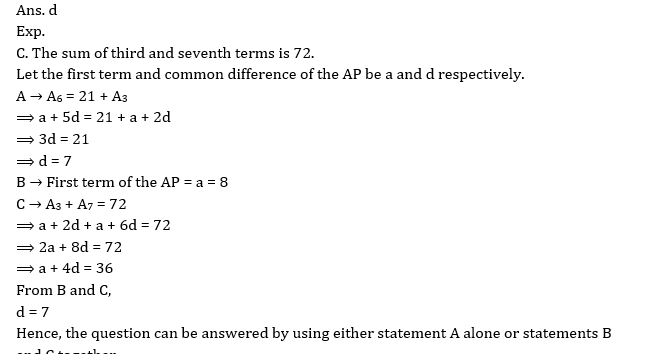2. What is the speed of the stream?
A. The boat goes 49 km downstream in 3.5 hours.
B. The ratio of the speed of boat in still water and the speed of stream is 6 : 1.
C. The boat goes 10 km more in downstream than in upstream in 2.5 hours.
(a) Either C alone or A and B together
(b) Only A and B together
(c) Only A alone
(d) Only B alone
(e) Only C alone3. What is the value of a two-digit number?
A. The ratio of the value of the number and the sum of the digits of that number is 4 : 1.
B. The sum of the original number and the number obtained after interchanging the digits is 66.
C. The number obtained after interchanging the digits is 18 more than the number itself.
(a) Only A and B together
(b) Only B and C together
(c) Only A and C together
(d) All the three together
(e) Any two of the three together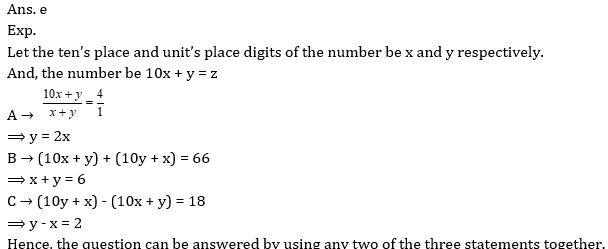4. Find out the length of a platform.
A. A Train moving with a speed of 72 km/h crosses the platform in 50 sec.
B. Ratio of the lengths of the train and the platform is 2 : 3.
C. The length of the train is 400 meters.
(a) Any two of the three together
(b) All the three together are not sufficient
(c) Only A and B together
(d) Only B and C together
(e) Only A and C together5. What is the ratio of the efficiency of a man and a woman?
A. 10 men are as efficient as 12 women.
B. 15 women can complete half of the work done by 25 men in same time.
C. A man is 20% more efficient than a woman.
(a) Only A alone
(b) Either A alone or C alone
(c) Any one of the three alone
(d) Only C alone
(e) Either A alone or B alone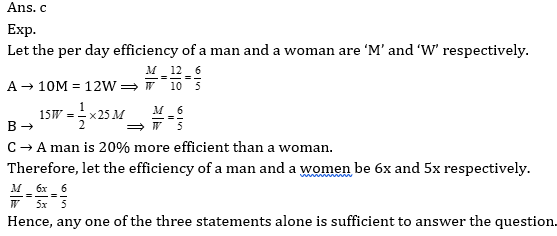6. Sam have some amount in his hand. He donated 30% of the amount, invested 40% of amount in a scheme which offers 15% p.a at SI, 10% of the amount lent to Jake but Jake returns only 6/7th of the amount he received and 20% of the amount is saved in the locker. Find the money Sam have initially if after one year he has Rs 20,880 in his hand.
(a) 31,500
(b) 24,500
(c) 35,000
(d) 28,000
(e) 27,300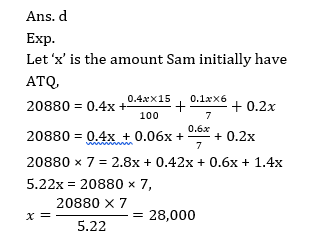7. Bus A and bus B start from point P and Q respectively and move towards each other. Buses cross each other after 4 hours. If the speed of Bus A increases and speed of Bus B decreases after every hour by 10 km/hr then find the distance between Point P and Q if average speed of buses is 70 km/hr.
(a) 560
(b) 490
(c) 420
(d) 630
(e) None of these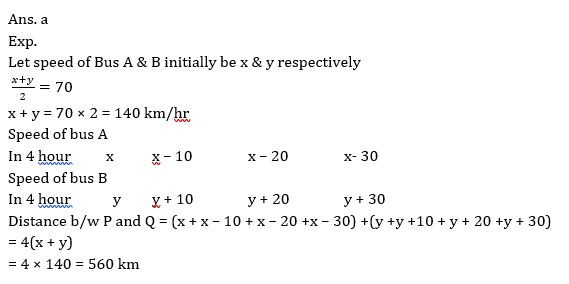8. The difference between the compound and the simple interest on a sum for 2 years at 10% per annum, when the interest is compounded annually, is Rs. 28. If the yearly interest were compounded half-yearly, the difference in the two interests will be :
(a) Rs. 44
(b) Rs. 28.35
(c) Rs. 43.41
(d) Rs. 43.29
(e) None of these9. If there is a country named zaamnagar population in that country is 18,000 out of which 50% are children and remaining are adults. Out of the adults in Zaamnagar 75% man and 50% women are married. How many people in Zaamnagar are married if it is known that there are no widows or widowers and every married person has only one spouse & no children are married?
(a) 3600
(b) 2100
(c) 2400
(d) 2700
(e) None of these10. A man gave 50% of his total savings of Rs. 168200 to his wife and divided the remaining sum among his sons Anthony and Brian of 15 and 13 years of age respectively. He divided it in such a way that each of his sons when they attain the age of 18 years, would receive the same amount, if interest rate is 5% compound interest per annum. The share of Brian was:
(a) Rs. 42050
(b) Rs. 40000
(c) Rs. 45000
(d) Rs. 45500
(e) None of these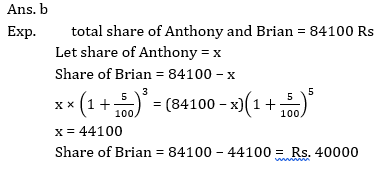### 2022 Preparation Kit PDF

#### Most important PDF’s for Bank, SSC, Railway and Other Government Exam : Download PDF Now

AATMA-NIRBHAR Series- Static GK/Awareness Practice Ebook PDF Get PDF here
The Banking Awareness 500 MCQs E-book| Bilingual (Hindi + English) Get PDF here
AATMA-NIRBHAR Series- Banking Awareness Practice Ebook PDF Get PDF here
Computer Awareness Capsule 2.O Get PDF here
AATMA-NIRBHAR Series Quantitative Aptitude Topic-Wise PDF 2020 Get PDF here
Memory Based Puzzle E-book | 2016-19 Exams Covered Get PDF here
Caselet Data Interpretation 200 Questions Get PDF here
Puzzle & Seating Arrangement E-Book for BANK PO MAINS (Vol-1) Get PDF here
ARITHMETIC DATA INTERPRETATION 2.O E-book Get PDF here
3